When you add three oranges to four oranges, you have seven oranges. When you have three oranges and you add four dogs, do you have seven? Sort of - but seven what? It's the same for fractions. As long as you're adding the same things then you'll be able to count them as if they were all oranges. Three tenths added to four tenths will equal seven tenths.

However, your child will be expected to come up with something a bit more advanced, namely adding with fractions that have different denominators (the number on the bottom), therefore they will have to carry out extra actions and not simply add.

If you have to add the following, you would need to change them into fractions sharing the same denominator.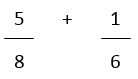The quickest way to the answer is to find the lowest number that both denominators fit into. If you don't, you can still do the sum but the answer will need to be simplified (see the walkthrough on simplifying fractions).

If in doubt, multiply together the two denominators as the answer will certainly be a number that both denominators fit into. In our example, it's 48. However, if you know your tables then you'll see that 8 and 6 both fit into 24, which is much less than 48. As the lowest common multiple of 6 and 8, it's going to give us the answer in a simpler form.

Now we must change the fractions into the new denominator, 24. Doing the inverse of the simplification process, all we need to do is multiply top and bottom by the same figure. Given that the number we need to reach on the bottom is 24, we must multiply both by 3.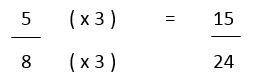Meanwhile, the other fraction had a denominator of 6. This means that it must be multiplied by 4 to reach 24. As with all equivalent fractions, we must DO TO THE BOTTOM WHAT WE DO TO THE TOP.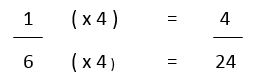Now we have converted both the fractions to the same denominator, we can simply add the numerators together.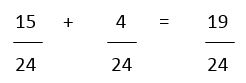This answer is in its simplest form. However, be aware that there might be some simplification needed.

## Subtracting Fractions

The subtraction of fractions works on the same premise as the addition - they need to be expressed in the same denominator. Otherwise you'll be unable to deal with them.

Let's look at an example: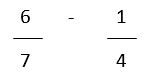The denominators are different so we must change them.

To make them the same, look at which number both 4 and 7 fit into. Times the two together and you get 28 - this also happens to be the lowest common multiple so that's what we'll stick with.

Now, change 6 over 7 into a fraction with 28 as its denominator. Work out how many times 7 fits in to 28 and multiply the numerator by the same number. You now have an equivalent (identical) fraction.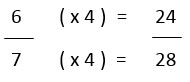Do the same to the other fraction: how many times does the denominator fit into 28? Answer 7, so multiply both top and bottom by this.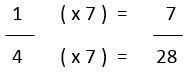Now we have two fractions with the same denominator, we can solve the sum. Treat it as if it were 24 oranges take away 7 oranges; the 'twenty-eighths' business shouldn't complicate matters. Simply take away the numerators.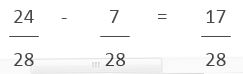Check that the fraction cannot be simplified and this is your answer, in its simplest form.